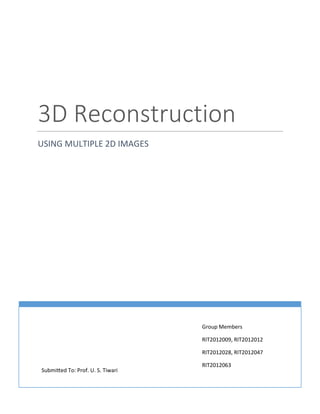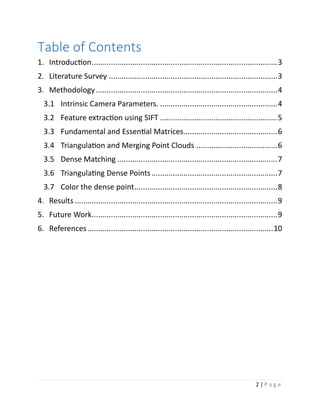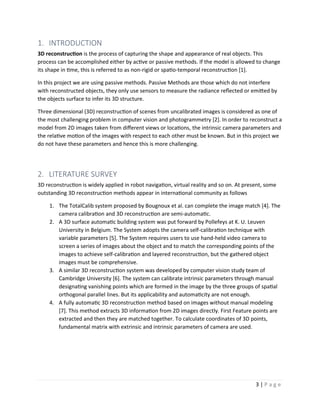Ce diaporama a bien été signalé.
Le téléchargement de votre SlideShare est en cours. ×

# 3D Reconstruction from Multiple uncalibrated 2D Images of an Object

Publicité
Publicité
Publicité
Publicité
Publicité
Publicité
Publicité
Publicité
Publicité
Publicité
PublicitéPublicité
Publicité
Chargement dans…3
×

## Consultez-les par la suite

1 sur 10 Publicité

# 3D Reconstruction from Multiple uncalibrated 2D Images of an Object

3D reconstruction is the process of capturing the shape and appearance of real objects. In this project we are using passive methods which only use sensors to measure the radiance reflected or emitted by the objects surface to infer its 3D structure.

3D reconstruction is the process of capturing the shape and appearance of real objects. In this project we are using passive methods which only use sensors to measure the radiance reflected or emitted by the objects surface to infer its 3D structure.

Publicité
Publicité

### 3D Reconstruction from Multiple uncalibrated 2D Images of an Object

1. 1. 1 | P a g e 3D Reconstruction USING MULTIPLE 2D IMAGES Group Members RIT2012009, RIT2012012 RIT2012028, RIT2012047 RIT2012063 Submitted To: Prof. U. S. Tiwari
2. 2. 2 | P a g e Table of Contents 1. Introduction.......................................................................................3 2. Literature Survey ...............................................................................3 3. Methodology.....................................................................................4 3.1 Intrinsic Camera Parameters. .......................................................4 3.2 Feature extraction using SIFT .......................................................5 3.3 Fundamental and Essential Matrices............................................6 3.4 Triangulation and Merging Point Clouds ......................................6 3.5 Dense Matching ...........................................................................7 3.6 Triangulating Dense Points...........................................................7 3.7 Color the dense point...................................................................8 4. Results ...............................................................................................9 5. Future Work.......................................................................................9 6. References.......................................................................................10
3. 3. 3 | P a g e 1. INTRODUCTION 3D reconstruction is the process of capturing the shape and appearance of real objects. This process can be accomplished either by active or passive methods. If the model is allowed to change its shape in time, this is referred to as non-rigid or spatio-temporal reconstruction . In this project we are using passive methods. Passive Methods are those which do not interfere with reconstructed objects, they only use sensors to measure the radiance reflected or emitted by the objects surface to infer its 3D structure. Three dimensional (3D) reconstruction of scenes from uncalibrated images is considered as one of the most challenging problem in computer vision and photogrammetry . In order to reconstruct a model from 2D images taken from different views or locations, the intrinsic camera parameters and the relative motion of the images with respect to each other must be known. But in this project we do not have these parameters and hence this is more challenging. 2. LITERATURE SURVEY 3D reconstruction is widely applied in robot navigation, virtual reality and so on. At present, some outstanding 3D reconstruction methods appear in international community as follows 1. The TotalCalib system proposed by Bougnoux et al. can complete the image match . The camera calibration and 3D reconstruction are semi-automatic. 2. A 3D surface automatic building system was put forward by Pollefeys at K. U. Leuven University in Belgium. The System adopts the camera self-calibration technique with variable parameters . The System requires users to use hand-held video camera to screen a series of images about the object and to match the corresponding points of the images to achieve self-calibration and layered reconstruction, but the gathered object images must be comprehensive. 3. A similar 3D reconstruction system was developed by computer vision study team of Cambridge University . The system can calibrate intrinsic parameters through manual designating vanishing points which are formed in the image by the three groups of spatial orthogonal parallel lines. But its applicability and automaticity are not enough. 4. A fully automatic 3D reconstruction method based on images without manual modeling . This method extracts 3D information from 2D images directly. First Feature points are extracted and then they are matched together. To calculate coordinates of 3D points, fundamental matrix with extrinsic and intrinsic parameters of camera are used.
4. 4. 4 | P a g e 3. METHODOLOGY Our 3D Reconstruction Pipeline consists of the following steps: 1. Intrinsic Camera Parameter. 2. Feature Extraction and Matching. 3. Getting Fundamental, Essential and [R|t] Matrix. 4. Triangulation to get points in 3D and merging them. 5. Dense Matching using Simple Propagation Technique. 6. Triangulating Dense Points. 7. Coloring and Display the Point Cloud created, in MeshLab or Blender. 3.1 Intrinsic Camera Parameters. For our project we are using same intrinsic matrix i.e. same camera and focal length for all images. We have neglected the effects of lens distortion (radial distortion). Assuming that optical center is at (0, 0) and focal length of camera is f. Our Intrinsic camera matrix will be K = 𝑓 0 0 0 𝑓 0 0 0 1 Input Images Extract Sift key points and its matches in consecutive frames Calculate Intrinsic Camera Parameter Extract Fundamental and Essential matrix Calculate [R|T] parameter for all frames Make graph of matched 3D points of frames Merge graph and Generate Sparse points Generate dense points Calculate image points for all dense points Coloring of Dense points Fig1: 3D Reconstruction Pipeline
5. 5. 5 | P a g e 3.2 Feature extraction using SIFT Given a feature in an image, what is the corresponding projection of the same 3D feature in the other image? This is an ill-posed problem and therefore in most cases very hard to solve. It is not clear that not all possible image features are good for matching. Often Points are used. Many interest point detector exist. In  Schmid ET. Al. concluded that the Harris corner detector gives the best results. Later more robust techniques developed, one of them is SIFT which is used in our project. Scale-invariant feature transform (or SIFT) is an algorithm in computer vision to detect and describe local features in images. The algorithm was published by David Lowe in 1999.  Scale space extreme detection - This is a filtering step that attempts to identify location and scales that are identifiable from different view of same object. We used difference of Gaussian (D) technique for this step. 𝐷(𝑥, 𝑦, 𝜎) = (𝐺(𝑥, 𝑦, 𝑘𝜎) − 𝐺(𝑥, 𝑦, 𝜎)) ∗ 𝐼(𝑥, 𝑦) Now we will compare each point of 𝐷(𝑥, 𝑦, 𝜎) with 8 neighbours at same scale, 9 in up and 9 in down scale and if value is minimum or maximum then this is extrema.  Key point Localization - Now we remove the low contrast point or poorly localized edge points. We calculate ration of largest to smallest eigenvector of Hessian matrix at location and scale of key point, if it is more than difference of Gaussian then rejected.  Orientation Assignment – We will calculate magnitude and direction of key points. Orientation is rounded-off to fit in 8 possible directions. 𝒎(𝒙, 𝒚) = √(𝑫(𝒙 + 𝟏, 𝒚) − 𝑫(𝒙 − 𝟏, 𝒚)) 𝟐 + (𝑫(𝒙, 𝒚 + 𝟏) − 𝑫(𝒙, 𝒚 − 𝟏)) 𝟐 𝜃( 𝑥, 𝑦) = tan−1 (𝐷(𝑥,𝑦+1)−𝐷(𝑥,𝑦−1)) (𝐷(𝑥+1,𝑦)−𝐷(𝑥−1,𝑦)) 𝑊ℎ𝑒𝑟𝑒 𝑚, 𝜃 𝑎𝑟𝑒 𝑀𝑎𝑔𝑛𝑖𝑡𝑢𝑑𝑒 𝑎𝑛𝑑 𝑜𝑟𝑖𝑒𝑛𝑡𝑎𝑡𝑖𝑜𝑛  Key point Descriptor – Key point descriptors typically uses a set of 16 histograms, aligned in a 4x4 grid, each with 8 orientation bins. This results to give feature vector containing 128 elements. We use K-D tree to match Key point descriptor of an image to another image. (In our Implementation - Number of matched key points should be more than 20). …..Equation 1 ….Equation 2 ….Equation 3
6. 6. 6 | P a g e 3.3 Fundamental and Essential Matrices We get fundamental matrix(F) by using 8 point algorithm and matched image features in two view, calculated by SIFT. We will calculate Essential matrix from Fundamental matrix using equation 4. 𝐸 = 𝐾 𝑇 𝐹𝐾 K Intrinsic camera matrix F Fundamental Matrix E Essential Matrix Extrinsic camera matrix from Essential matrix - : Singular value decomposition of Essential matrix is used to find position matrix of camera for different views. Set Position of one camera at origin ( 𝑅|𝑇1 = [𝐼|0]) .We will find second position matrix ( 𝑅|𝑇2) for another frame. We can write 𝐸 = 𝑈𝐷𝑉 Here 𝑊 = 0 −1 0 1 0 0 0 0 1 and U & V are orthogonal matrix. From above representation of E we will get two rotation matrix and one translation vector with different sign. We have 4 different rotation-translation (R|T) matrix. We will choose best R|T matrix for 2nd view. The above method can be used only for initial frame. For rest of the frame we have position matrix of previous frame. So we will calculate position matrix by 3D point (X) obtained from previous frame. 𝑥 = 𝑃𝑋 Here x is image point and X is corresponding 3D point which are known, P can be calculated. 3.4 Triangulation and Merging Point Clouds Triangulation refers to the process of determining a point in 3D space given its projections onto two or more images . We have pairs of adjacent images in sequence and their essential matrix. Now for every pair of image we will do triangulation and store them in the structure, in sequence. Bundle Adjustment is done over graph in order to refine the 3D coordinates describing the scene geometry as well as the parameters of relative motion and optical characteristics of camera which is used to acquire the images. Different Set of 3D calculated are merged together in next step. Merged collection of all points is plotted in mesh lab which give just the glimpse of original object thus we requires ….Equation 4 ….Equation 5 ….Equation 6
7. 7. 7 | P a g e further processing for better 3D representation. This merged Collection of points is also called Sparse Point Cloud. 3.5 Dense Matching Acquisition of less number of 3D points is the reason of poor results in sparse Point Cloud, thus we need large number of points present in the neighbors of currently acquired 3D points to get continuous 3D object. This is achieved through dense matching. We use ZNCC score with simple matching propagation in order to achieve the dense mapping of consecutive images. Algorithm is as follows: For each pair of consecutive images: 1) First of all, change both the images into its corresponding gray value images. 2) Calculate the ZNCC value for both the images. 3) Take the central value as the current matched features in both images which is calculated in previous step. 4) Propagation: Match the neighbors of central pixels in both the images according to the maximum ZNCC score between the neighbors (evaluated by ZNCC) and propagate only in the match-able area. 𝑍𝑁𝐶𝐶(𝑥1, 𝑥2) = ∑ ((𝐼(𝑥1 + 𝑖) − (𝐼̅( 𝑥1))) (𝐼(𝑥2 + 𝑖) − (𝐼̅( 𝑥2 + 𝑖))𝑖=𝑛 𝑖=0 √∑ ((𝐼(𝑥1 + 𝑖) − (𝐼̅( 𝑥1))2 ∑ ((𝐼(𝑥2 + 𝑖) − 𝐼̅( 𝑥2)))2𝑖=𝑛 𝑖=0 𝑖= 𝑛 𝑖=0 Where 3.6 Triangulating Dense Points After Dense Matching, we need triangulate newly generated dense points. 1. Firstly, we do the estimation of 3D point(X) from image matches and camera matrices. 2. Estimation is done by using the Non-Linear Approach. 3. For this estimated 3D point, we find the coordinates of this point on the image plane. 4. Find the error between the initial dense point and the point came in third step. 𝑥1, 𝑥2 Central Points in Image1 and Image2. (𝑥1 + 𝑖), (𝑥2 + 𝑖) Neighboring Points 𝐼 Average gray Value Image ….Equation 7 ….Equation 8
8. 8. 8 | P a g e 5. Calculate the direction vectors from camera center (C) to 3D point’s i.e. (X-C). 6. Change this direction vectors into unit vectors then calculate the angle between this unit vectors. 7. By using this condition, we test that point(X) is acceptable as 3D or not. (𝑋 − 𝐶). 𝑅(3, : ) 𝑇 > 0 𝑤ℎ𝑒𝑟𝑒 𝐶: −𝑅 𝑇 𝑡 𝑅(3, : ) 𝑇 ∶ 𝑉𝑖𝑒𝑤 𝐷𝑖𝑟𝑒𝑐𝑡𝑖𝑜𝑛 𝑣𝑒𝑐𝑡𝑜𝑟 3.7 Color the dense point After finding the 3D point, we need to color those points. Coloring of these points is done in two steps:-  Firstly, for each 3D point we find the corresponding point in the images by using 𝒙 = 𝑷𝑿  Set the color of 3D point is equal to color of the corresponding point in Image. ….Equation 9 ….Equation 6
9. 9. 9 | P a g e 4. RESULTS 5. FUTURE WORK A lot of Scope is present for Improvement. 1. Removing performance bottleneck. 2. Improving Feature Detection. 3. Creating Mesh from Point Cloud. 4. Applying Texture Mapping. 5. On the Fly Calculation of Intrinsic Camera Matrix. 6. Incorporating Improvements to Handle Radial Distortion and Skewness. Fig2: Dense Point Cloud View 1 Fig3: Dense Point Cloud View 2 Fig4: Dense Point Cloud View 3 Fig5: Original Image with Key points
10. 10. 10 | P a g e 6. REFERENCES  3D-Reconstruction: http://en.wikipedia.org/wiki/3D_reconstruction  M. Pollefeyes, R. Koch, M. Vergauwen and L. Van. “Automated reconstruction of 3D scenes from sequences of images”, ISPRS journal of photogrammetry & remote sensing 55, 251-267, 2000.  Lowe, David G. (1999). "Object recognition from local scale-invariant features". Proceedings of the International Conference on Computer Vision 2. pp. 1150–1157.  Bougnoux S. and Robert L., “A fast and reliable system for off-line calibration of images sequences”, Proc. Compute Vision and Pattern Recognition, Demo Session, 1997.  Polleleys M., “Self-calibration and metric 3D reconstruction from uncalibrated image sequences”, Ph.D. Thesis, Katholieke Universiteit Leuven, Heverlee, 1999.  Cipolla R., Robertson D. P. and Boyer E.G., “Photobuilder-3Dmodels of architectural scenes from uncalibrated images”. Proc. IEEE International Conference on Multimedia Computing and Systems, Firenze, volume 1, pp.25-31, June, 1999.  Jiang Ze-tao, Zheng Bi-na, Wu Min, Wu Wen-huan, “A Fully Autmatic 3D Reconstruction Method Based on Images”, Computer Science and Information Engineering, 2009 WRI World Congress, volume 5, pp. 327-331, March-April, 2009.  YUAN Si-cong; LIU Jin-song, “Research on image matching method in binocular stereo vision. Computer Engineering and Applications”, 2008，44 (8): pp. 75-77.  Triangulation: http://en.wikipedia.org/wiki/Triangulation ===================== Remarks ===================